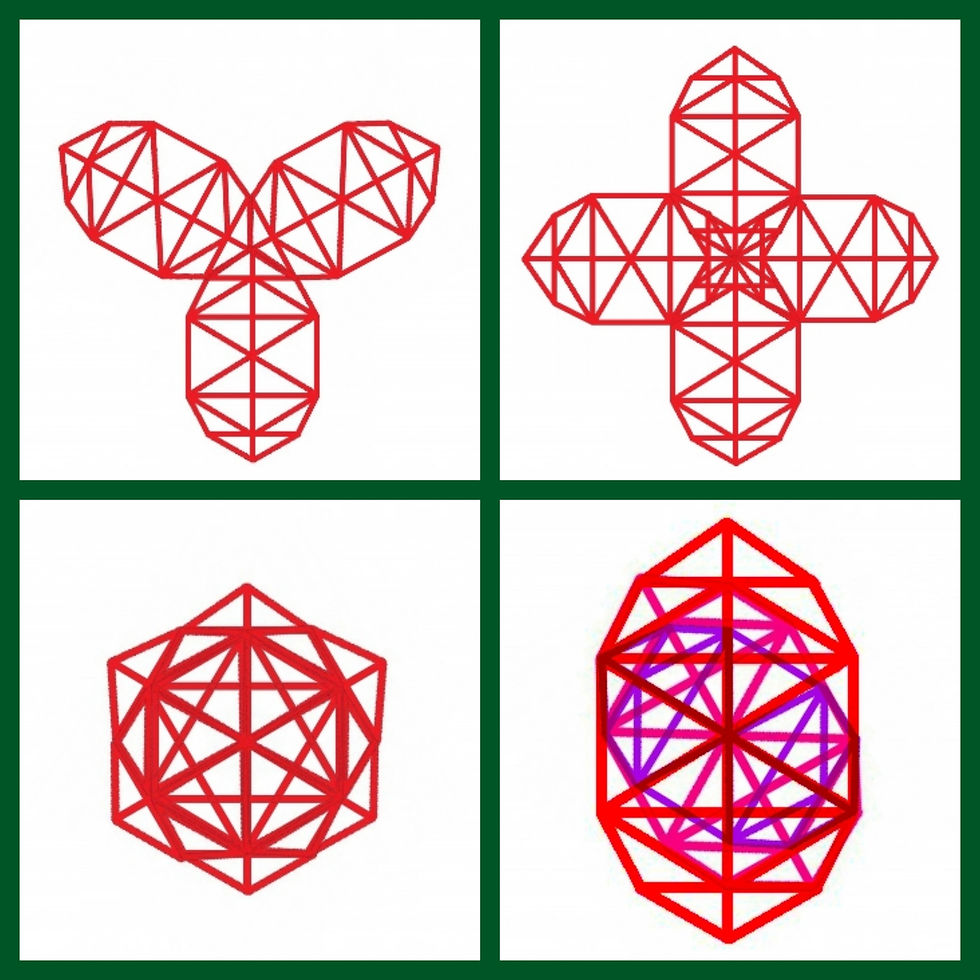top of page
Search

# Hexaflor and Hexatree(13 Sephirot-15 dimensional Kabbalistic tree of life) part 1

The Doubling-Fibonacci circles looks like the torus cross section as I have mentioned before, and this structure (when complete) forms waves, and when its multiplied by 3 it forms the fruit of life(flower of life) which therefore is based on phi proportions and forms the fractaling star tetrahedron so it can be formed out of 6(12) Fibonacci spirals, which I have already shown with the Fibonacci spiral forming the triangle encoding harmonics(in the form of waves), this is the hexaflor/fractaling nesting hexagon and when this geometry is nested inside of itself it forms the (64) tetrahedron grid/flower of life, the hexaflor as you can see looks like a torus.

The hexaflor, as I said, is constructed out of 6-12 Fibonacci spirals, this structure forms and is formed out of nesting hexagons which forms the flower of life/64 tetrahedron grid and this structure is a torus and the torus forms and is formed out of the flower of life.I wanted to calculate the growth rate of the hexaflor/fractaling hexagon, I measured six sides by zooming into the screen and using a ruler to measure them and I tried to get one of the side equal to 10cm, but I couldn't but I did get one side equal to 9. I then calculated the growth rate in two ways, the first way I used the six measured sides to calculate the growth as you can see in the picture and the second way I calculated the growth rate by simplifying the ratio 9:7.5 and I got 1.2 so the growth rate is or is around 1.2. The simplified ratio is interesting because I've come across 0.8333, 5/6 and 6/5 in my research into sacred geometry before and I've come across 0.8333 before in string theory in the form of (1+2+3+4+...=)-1/12. To get the numbers in the sequence you calculate 1.2^n, with n being any whole number.

A part of a hexagon with 1cm sides contains a hexagon with sides roughly equal to 0.8cm:An interesting number in this sequence is the 27th and -27th number:

• 1.2^27=137

• 1.2^-27=0.0072

• 1/137=0.0072When the Kabbalistic tree of life is doubled it forms the 13 middot which is basically the 13 sephirot kabbalistic tree of life, when I overlayed it over the hexaflor I noticed the center which lines up with the number 6 forms the hexagon and the hexaflor expands out of it so I recreated the 13 sephirot tree based on the 3D tree of life(16 sephirot) and it fit on the hexaflor so the 13 sephirot tree expands out of the hexaflor and when it fractals it forms the hexaflor/fractaling nesting hexagon so I named the 13 sephirot tree the hexatree.When the hexatree is tripled it forms the (nesting) hexagon and this can also be seen when the tree fractals forming the Fibonacci spiral-Hexaflor, the hexatree can be joined with two other hexatrees to form a triangular structure, the hexatree when joined to three other hexatrees forms a 4-fold structure which forms 4 expanding triangles which can be seen in the fractaling hexatree and when there overlayed it can be seen, this structure also forms the prime number cross.This 4-fold hexatree structure is called the 4-hexatree and can be derived from the 12-hexatree a 12-fold hexatree which is contained within the hexatree.When the hexaflor/hexatree nests/fractals within itself it forms the 64 tetrahedron grid/flower of life because the hexagonal numbers are encoded in the hexaflor but as can be seen there are missing hexagons which are formed when the hexagons are doubled, rotated and nested and this also fits in the hexaflor/double hexaflor, which is a torus, which as you can see plots out the points of the flower of life which the torus is formed out of when it spins/doubles/nests/fractals which is all formed out of the Binary-Trinary sequence. Lets look at the cube(istic matrix) now, since the hexagon doubles and rotates we'll have to do the same with the cube but we can't but we can if we were to use its dual the octahedron which would form a fractaling cube octahedron nesting, within metatrons cube, forming the vector equilibrium/tetrahedron grid fractal which is also forms a torus and is formed within the hexatree fractal.The Fibonacci spiral/(double) hexaflor(torus) lines up with the golden angle 137.5(the fine structure constant)(?)I thank you for reading, part 2 will be about the dimensions of the hexatree and will hopefully be released soon.